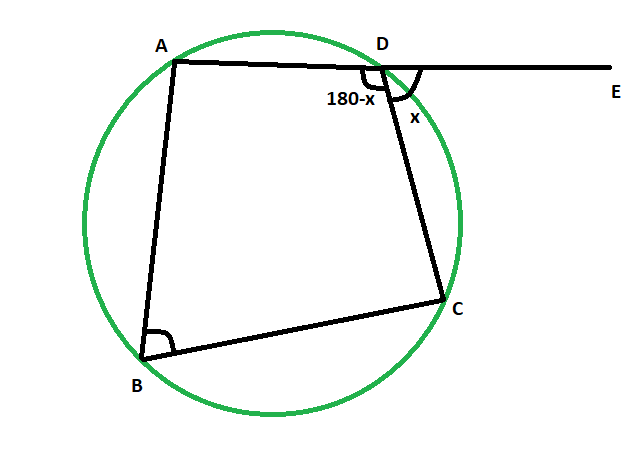# Exterior angle of a cyclic quadrilateral when the opposite interior angle is given

Given cyclic quadrilateral inside a circle, the task is to find the exterior angle of the cyclic quadrilateral when the opposite interior angle is given.

Examples:

```Input: 48
Output: 48 degrees

Input: 83
Output: 83 degrees
```Approach:

• Let, the exterior angle, angle CDE = x
• and, it’s opposite interior angle is angle ABC
• as, ADE is a straight line
• so, angle ADC = (180-x) degrees
• since, opposite angles of a cyclic quadrilateral are supplementary,
• angle ABC = xBelow is the implementation of the above approach:

## C++

 `// C++ program to find the exterior angle ` `// of a cyclic quadrilateral when ` `// the opposite interior angle is given ` ` `  `#include ` `using` `namespace` `std; ` ` `  `void` `angleextcycquad(``int` `z) ` `{ ` `    ``cout << ``"The exterior angle of the"` `         ``<< ``" cyclic quadrilateral is "` `         ``<< z << ``" degrees"` `<< endl; ` `} ` ` `  `// Driver code ` `int` `main() ` `{ ` `    ``int` `z = 48; ` `    ``angleextcycquad(z); ` `    ``return` `0; ` `} `

## Java

 `// Java program to find the exterior angle ` `// of a cyclic quadrilateral when ` `// the opposite interior angle is given ` ` `  `import` `java.io.*; ` ` `  `class` `GFG  ` `{ ` ` `  `static` `void` `angleextcycquad(``int` `z) ` `{ ` `    ``System.out.print( ``"The exterior angle of the"` `        ``+ ``" cyclic quadrilateral is "` `        ``+ z +``" degrees"``); ` `} ` ` `  `// Driver code ` `public` `static` `void` `main (String[] args)  ` `{ ` `        ``int` `z = ``48``; ` `    ``angleextcycquad(z); ` `} ` `} ` ` `  `// This code is contributed by anuj_67.. `

## Python3

 `# Python program to find the exterior angle ` `# of a cyclic quadrilateral when ` `# the opposite interior angle is given ` ` `  `def` `angleextcycquad(z): ` `    ``print``(``"The exterior angle of the"``,end``=``"");  ` `    ``print``(``"cyclic quadrilateral is "``,end``=``"");  ` `    ``print``(z,``" degrees"``); ` ` `  `# Driver code ` `z ``=` `48``; ` `angleextcycquad(z); ` ` `  `# This code is contributed by 29AjayKumar `

## C#

 `// C# program to find the exterior angle ` `// of a cyclic quadrilateral when ` `// the opposite interior angle is given ` `using` `System; ` ` `  `class` `GFG  ` `{ ` ` `  `static` `void` `angleextcycquad(``int` `z) ` `{ ` `    ``Console.WriteLine( ``"The exterior angle of the"` `        ``+ ``" cyclic quadrilateral is "` `        ``+ z +``" degrees"``); ` `} ` ` `  `// Driver code ` `public` `static` `void` `Main ()  ` `{ ` `        ``int` `z = 48; ` `    ``angleextcycquad(z); ` `} ` `} ` ` `  `// This code is contributed by anuj_67.. `

Output:

```The exterior angle of the cyclic quadrilateral is 48 degrees
```

Attention reader! Don’t stop learning now. Get hold of all the important DSA concepts with the DSA Self Paced Course at a student-friendly price and become industry ready.

My Personal Notes arrow_drop_upCheck out this Author's contributed articles.

If you like GeeksforGeeks and would like to contribute, you can also write an article using contribute.geeksforgeeks.org or mail your article to contribute@geeksforgeeks.org. See your article appearing on the GeeksforGeeks main page and help other Geeks.

Please Improve this article if you find anything incorrect by clicking on the "Improve Article" button below.

Improved By : vt_m, 29AjayKumar

Article Tags :
Practice Tags :

Be the First to upvote.

Please write to us at contribute@geeksforgeeks.org to report any issue with the above content.